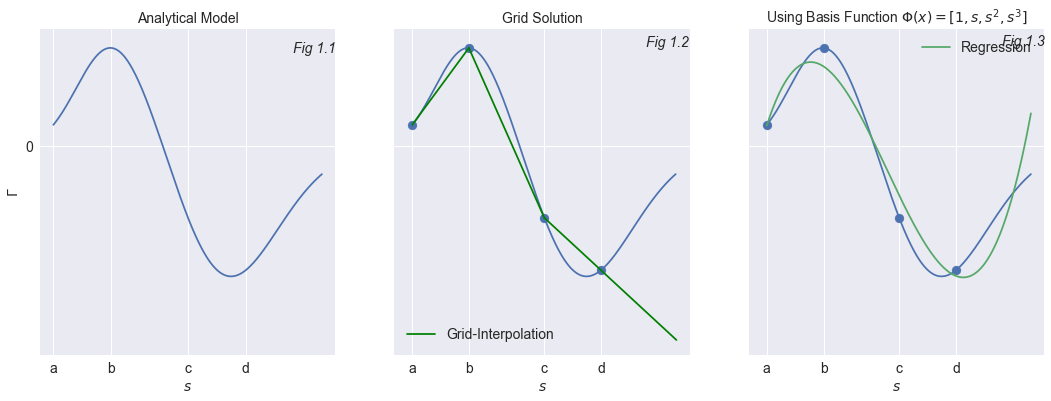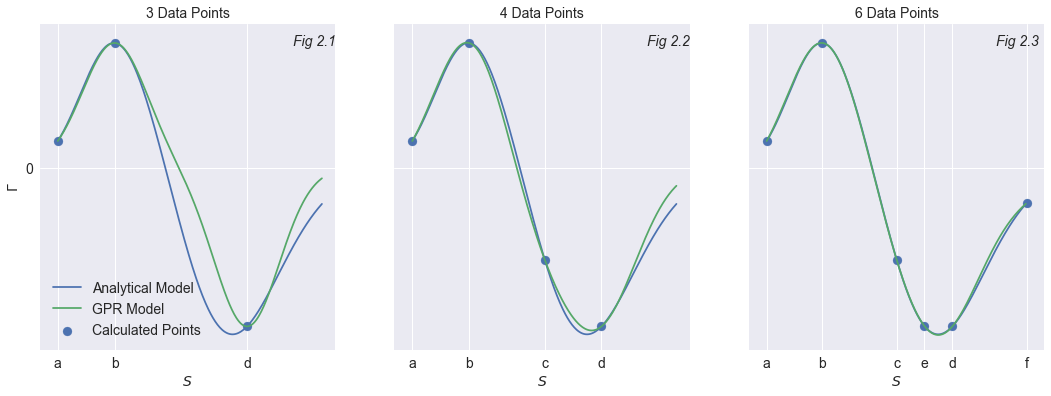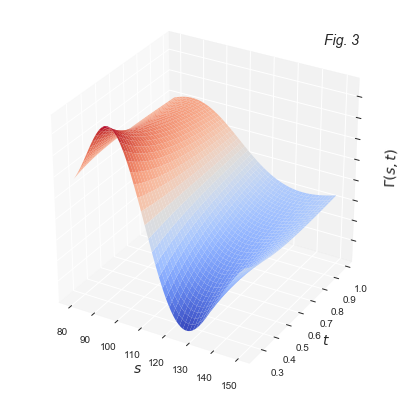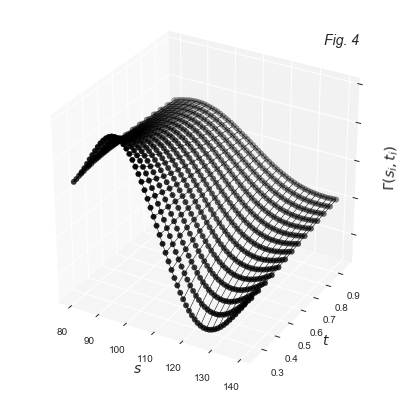May 28, 2018

# Quant vs. Machine

### Boosting Derivatives Pricing by Machine Learning

In the derivatives world, zillion computations need to be done on a daily basis. Models need to be calibrated. Derivative instruments need to be priced. Hedge positions need to be calculated. Risk management indicators need to be determined. With the “Fundamental Review of the Trading Book” (FRTB) luring around the corner, an even larger computational challenge is coming to every bank's direction. The December 2019 deadline is approaching. Fortunately machine learning can help as we illustrate in this contribution. For once this is a practical application of machine learning, not yet another trial to beat the stock market with deep learning predictions.
In this contribution, we show how one can deploy machine learning techniques in the context of traditional quant problems. We illustrate that for many classical problems, we can arrive to speed-ups of several orders of magnitude by deploying machine learning techniques based on Gaussian process regression. The price we have to pay for this extra speed is some loss of accuracy. However, we show that this reduced accuracy is often well within reasonable limits (for example within bid-ask spread) and hence very acceptable from a practical point of view.### Introduction

Deep Learning is indeed a hot topic in finance; there is no place to hide from it. Neural network models have gradually found their way onto trading desks. Quants changed business titles and became self-proclaimed data scientists. Many have been taking the bull by the horns and are using deep learning methods in their goal to forecast financial markets. We wish them good luck. Some are feeding their models with time series, while some of the other treasury-hunters go a step further and use unstructured data such as news feeds and website content into their prediction models. This is where sentiment analysis comes into the picture. In this contribution we focus on derivatives pricing using these new techniques. We could also rely on a neural network to achieve this goal. After all, the universal approximation theorem states that simple neural networks can represent any bounded continuous function (George Cybenko 1989). But we deliberately step in a different direction. Our approach is one where we rely on a machine learning technique to price a portfolio of derivatives extremely fast. We train our model to understands derivatives pricing and make predictions once trained.

### Pricing exotic derivatives

Getting a theoretical value for an exotic option can be computationally intensive. More than often Monte Carlo techniques have to be put at work to get the job done. The nature of most complex derivatives is also such that it is wrong to price them in a Black-Scholes setting. This is where stochastic volatility models such as the Heston model come into the picture. The pricing model becomes more realistic but now requires extra parameters to be calibrated to match a particular volatility surface derived from listed option prices. As a consequence, the computation time is driven upwards. Although one has in the last decades invested lots of research in speeding up the calculations, many institutions still face the limitations. Even if a calculation is possible in a fraction of a second, it can still be problematic if these calculations need to be repeated almost continuously on thousands of option prices and thousands of underliers. Real-time values are hence not always feasible. The whole thing becomes more precise but slower and slower...
Trading desks are more than often making a dangerous compromise between speed and accuracy. Auditors have a similar challenge. They have to make an independent valuation of the value of a derivatives portfolio. Here again speed is key and there is the eminent risk that corners will be cut in order to get the job done in time. Speed can be a bad advisor.
Let us consider a simple example of a long position in a cliquet call option of which we want to study the gamma $\Gamma$ as a function of the share price $s$. As illustrated in Fig 1.1, the gamma of this option is double-signed. As the share price increases the $\Gamma$ flips from positive to negative. Cliquet options are priced with a Heston model emphasizing the need to rely on a stochastic volatility model to deal with such an option. The smooth representation of $\Gamma(s)$ in Figure 1.1 requires a large computational workload. As a quick fix, one often proposes two alternative approaches:

• Grid Solution

For a selection of share price values $s \in [a, b, c, d]$, the value of $\Gamma(s)$ is calculated. These calculations take place overnight and the results are stored in a repository. Intraday, the value of $\Gamma(s)$ is obtained via linear interpolation in the grid. The solution in Figure 1.2 illustrates how the bias between the estimate $\hat{\Gamma}$ and the analytical solution $\Gamma$ can be improved when using a grid with a higher density. But again, this comes with an additional computational cost.

• Regression Model

A regression model is similar to a grid solution, since one starts with the calculation of the value of $\Gamma(s)$ for different values of $s\in [a,b, c, d]$ in a grid. Of course the nature of the function $\Gamma(s)$ does not facilitate the use of a linear interpolation. The function is too "wobbly" for this. This is where our grid solution derailed completely and why basis functions are introduced. The model inputs $s_1,s_2,\ldots,..s_N$ are organized into a design matrix $X$. This matrix has in our case 4 rows ($N=4$) and 1 column.
$X$ is transformed from a column vector of size $N \times 1$ to a matrix with dimension $N\times q$:
$$X\rightarrow \Phi(X) \in \mathbb{R}^{N\times q}$$.

In this case we are using a polynomial of order 3 to replicate the analytical solution:
$$\begin{equation} \begin{array}{lll} X &=& [1 , s , s^2 , s^3]\\ &=&\left[ \begin{array}{llll} 1&s_1&s_1^2&s_1^3\\ \vdots&&&\vdots\\ 1&s_N&s_N^2&s_N^3\\ \end{array}\right]\\ \end{array} \end{equation}$$
For each row $\mathbf{x_i}$ in the design or data matrix $X$ , there is a corresponding output $y_i$. Estimated outputs are obtained in the regression model as :
$$y_i= \Gamma(s_i)= w_0+w_1s_i+w_2s_i^2+w_3s_i^3$$
The result of this interpolation is illustrated in Figure 1.3. The choice of the basis function $\Phi(s)$ is far from straightforward. Where in our example a polynomial of order 3 seemed a logical choice, a similar basis function might fail for different parameters.### Machine Learning

The problem with the interpolation was the choice of the basis function $\Phi()$. This is where a machine learning technique such as Gaussian Process Regression (GPR) is going to take over. This learning model is non-parametric since it requires no parameters or statistical conditions on the population. The data will decide for themselves what the optimal model $y=f(X)$ is going to be. Let's start with a simple example using the same cliquet option. In Figure 2.1, 3 points ($a,b,c$) are selected. For these inputs the analytic solution is calculated. The GPR model has visibly not a satisfatory result and requires more inputs.

Figure 2.2 illustrates that adding a single extra data-point $[c, \Gamma(c)]$ gets us in the right direction. The model has one extra point in the training set and the outcome clearly improves. A further increase to 6 data points leads to an even better fit as shown in Figure 2.3.
This summarizes in a nutshell the whole procedure:

• GPR-Training

For a large grid of pricing parameters and model parameters, the option prices and sensitivities such as gamma, vega, etc..are calculated and stored into a repository. This data structure is summarized into a dedicated Kernel matrix $K$. Once the Kernel is calculated, the training of the model is accomplished.

• GPR-Pricing

Starting from the kernel $K$ and after applying GPR-related algebra to it, any new pricing of the derivative structure takes places extremely fast.

</ul>The results in the Figure above , combined with the gain in pricing speed, turned us into big fans of this GPR-technique.

### Adding more dimensions to GPR

So far the $N$ inputs of the pricing model were gathered into a design matrix with only one column containing the share prices $s_i$. The share price $s$ was the only parameter. The cliquet call option for which we developed the pricing model has more parameters that are driving its value. One can make a distinction between market parameters and model parameters:
• Market Parameters
In a Black-Scholes setting we have to take the share price, the volatility , the interest rate-level, the dividend yield and the time to maturity into account. Since the cliquet model requires a stochastic volatility model such as Heston, 5 extra parameters will have to be added to the data or design matrix $X$.
• Model Parameters
The model parameters represent the characteristics of the option for which we want to obtain the price, delta, gamma, vega,etc...The cliquet call option has a strike, a local and global cap, a local and global floor, an equity participation rate, a number of cliquet periods and the length of each of the cliquet periods.

Taking all of these $p$ parameters into account reshapes $X$ from a column vector into a matrix with dimension $N \times p$. We are no longer training GPR on a column vector but on a matrix.
Our training data consists of a set containing $N$ observations $(X,\mathbf{y})= \{(x_i,y_i) \mid i=1,\ldots,N \}$As explained above the first step is the training of the model. For a training set of $N$ observations across different share price levels $s$ and maturities $t$, the function value is calculated.
Each row $\mathbf{x_i}$ in the data matrix $X$ has two entries: a share price level and time to maturity : $\mathbf{x_i} = [s_i,t_i]$. Each input value $\mathbf{x_i}$ has a corresponding calculated function value $y_i$. In our case this is the Gamma of a cliquet call option: $y_i = \Gamma(s_i,t_i)$

An example of a training grid is illustrated in the figure below:Once trained, the GPR model can now be used to calculate the function value $y$. In our case, this was the Gamma of a capped cliquet call option. The training is the only time consuming component of GPR. But each option type, fortunately only has to be trained once. The next time one is dealing with a cliquet option perhaps with a different cap level or a different parameters for the underlying, one can still benefit from earlier training work.

### Just Another Interpolation Method ?

It is not because the function is trained on a pre-determined grid of inputs $X$ that GPR should be seen as yet another interpolation method on this grid. GPR is a non-parametric approach that will deliver the distribution of functions $f(X)$ that are consistent with the observed data $(X,y)$. This is where a large cloud of input-and output values $(\mathbf{x_i},y_i)$ can be put at work to find a function value $f(\mathbf{x_*})$ for a new input $\mathbf{x_*}$. As we will discuss later, GPR uses a covariance matrix to ensure that values $\mathbf{x}$ that are close together in the input space will produce outputvalues that are also going to be close together.
We will explain how this large amount of information is summarized using kernel functions and how all of this leads to a fast and precise calculation of function values for new derivatives.

### Gaussian Process Regression Explained

#### Step 1: Training the Model

We start with the assumption that the coefficients $w$ in our regression model are following a multivariate normal distribution with mean 0 and covariance $\Sigma_w$. This is a first major difference with most regression techniques where the values $w$ are considered fixed.
$$w \sim N(0,\Sigma_w)$$
The weight vector $w$ remains the same for every input vector $\mathbf{x_i}$ and as such every forecast $\hat{y_i}=f(\mathbf{x_i})$ is a linear combination of jointly normally distributed variables $w$. As a consequence the response $f(X)$ can be described as a Gaussian process: $$f(x) = [\mathbf{x_1},\ldots,\mathbf{x_N}]$$
This process $f(X)$ is completely described by its mean function $m(X)$ and a covariance or kernel function $K(X,X)$. One often assumes a zero mean function:

$$f \sim N\left(0,K(X,X)+\sigma_{\epsilon}^2I_N\right)$$
Where the covariance matrix is defined as : $$\begin{equation} K(X,X)= \left[ \begin{array}{ccc} k(\mathbf{x_1},\mathbf{x_1})&\ldots&k(\mathbf{x_1},\mathbf{x_N})\\ \vdots&\vdots&\vdots\\ k(\mathbf{x_N},\mathbf{x_1})&\ldots&k(\mathbf{x_N},\mathbf{x_N})\\ \end{array} \right] \end{equation}$$

Each of the functions $k(\mathbf{x_i},\mathbf{x_j})$ is a kernel function. Here plenty of choices are available. In our example we used a radial basis function (RBF) which is defined as follows:

$$k(\mathbf{x_i},\mathbf{x_j}) = \alpha \exp{\left(- \sum_{k=1}^{p} \left(\frac{\mid x_{ik}-x_{jk}\mid }{\gamma_k}\right)^\beta\right)}+\sigma$$
Looking for an intuitive explanation for $k(\mathbf{x_i},\mathbf{x_j})$, we could consider its value as some kind of a distance metric between the two data-points $\mathbf{x_i}$ and $\mathbf{x_j}$ in of our dataset $X$. At first this looks like a step in the wrong direction since our input matrix sees its dimension increased from $N \times p$ to $N \times N$ when introducing the kernel matrix $K(X,X)$.

A critical reader will also observe that while trying to find an elegant and fast procedure to price derivative instruments, the solution to our problem looks now even further away! Not only did the dimensionality of the problem increase, but one also notices the introduction of new parameters: $\alpha, \gamma_1, \ldots,\gamma_p,\sigma$. Finding the optimal values of these hyperparameters is an integral part of the Gaussian Process Regression approach. But again, one only needs to do this once! Building the dataset $X$, calculating $K(X,X)$ and determining the hyperparameters might be computational intensive; but once trained, finding the value $f(\mathbf{x})$ is extremely fast and easy.

#### Step2: Making Predictions

Consider a new datapoint $\mathbf{x_*}$ for which we want to predict the function value $y_*=f(\mathbf{x_*})$. Given the GPR model we can write that: $$\begin{equation} \left[ \begin{array}{l} \mathbf{y}\\ y_* \end{array} \right]\sim N\left(0,\left[ \begin{array}{ll} K(X,X)+\sigma_{\epsilon}^2I_N &&K(X,\mathbf{x_*})\\ K(\mathbf{x_*},X)&&K(\mathbf{x_*},\mathbf{x_*}) \end{array} \right]\right) \end{equation}$$
Based on the new input $\mathbf{x_*}$,a training set $X$ and corresponding output $\mathbf{y}$, the value of $y_*$ follows a normal distribution:
$$y_*\mid \mathbf{x_*},X,\mathbf{y} \sim N(\mu,\Sigma)$$ with
$$\mu = K(\mathbf{x_*},X)[K(X,X)+\sigma_{\epsilon}^2I_N]^{-1}\mathbf{y}$$ and $$\Sigma = K(\mathbf{x_*},\mathbf{x_*})-K(\mathbf{x_*},X)[K(X,X)+\sigma_{\epsilon}^2I_N]^{-1}K(\mathbf{x_*},x)$$ The predicted value $\hat{y_*} = E[y_*]=\mu$### RiskConcile's Implementation of GPR

#### 1.Storing $K(X,X)$ on cloud-repository

Of the two steps, training and predicting, the first one represents a heavy computational burden. Building the matrix $K(X,X)$ requires a repricing of the financial instrument across all possible combinations of values for the model and market parameters. For complex models requiring Monte Carlo, this will be time consuming. The results of each pricing $\mathbf{y}$ and the corresponding input $X$ are captured and stored in a database. Here we have chosen a cloud based solution. To speed up the pricing, the workload is split amongst different calculation servers.

#### 2.Hyperparameter optimisation

The parameters specifying the chosen kernel function need to be optimized. Given the training data $X$ and the corresponding calculated output $\mathbf{y}$ the log-likelihood is used to determine the optimal choice for the hyperparameters. The larger the value of the log-likelihood, the better the choice. Given the non-linearity of this log-likelihood function, gradient-based optimizers will be used.

#### 3.Solving $K(X,X)^{-1}$

Calculating the value $\mu$ to find a prediction for our option price, gamma, vega,etc... is now extremely fast if and only if $K(X,X)^{-1}$ is calculated beforehand. It would not be good practice to calculate the inverse of $K(X,X)$ in each prediction. Inverting the matrix $K(X,X)$ of size $N\times N$ is the final computational challenge to take. For complex derivatives priced using models having a wide range of values for the different market parameters, the size of $K(X,X)$ can get too large for proceed to a standard matrix inversion on a computer. Moreover, in the hyperparameter optimization step $K(X,X)^{-1}$ needs to be calculated for each intermediate calculation. RiskConcile developed a different approach to this problem. The number of points $N$ in the training data is no longer a constraint.

#### 4.Deployment

RiskConcile is doing the heavy lifting. For a wide variety of options, the kernel $K(X,X)$ and its inverse $K(X,X)^{-1}$ have been calculated. Users of our web-infrastructure can now pull quasi real-time option prices into their applications (Excel, MatLab, Python, R,...) .

### Conclusion

We have tested GPR as machine learning technique on a wide range of complex derivative structures. GPR was effectively used to train a model how to price exotic options. Starting from training data $X$ and corresponding outputs $\mathbf{y}$, the gain in calculation time was enormous! For barrier options for example, while using a Variance Gamma model, the pricing turned over 1000 times faster!